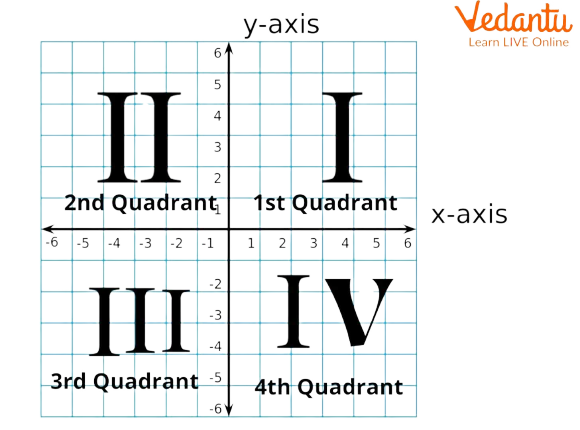Courses
Courses for Kids
Free study material
Free LIVE classes
More

# Cartesian Form of Vector - JEE Important TopicsLIVE
Join Vedantu’s FREE Mastercalss

## An Introduction to Cartesian Form

The geometric entities in the cartesian plane can be represented on a three-dimensional plane, this representation is termed as cartesian form. It is represented in a three-dimensional form via X, Y, and Z axes. Along with this, we can also represent a point, a line, or a plane in a cartesian form. Let’s dig deeper into cartesian form and equations.

## Cartesian Coordinates

Cartesian coordinates assist in discovering the precise spot of a factor in a 3D aircraft. The Cartesian coordinates of a factor are a couple of numbers which can be both in three dimensions or two dimensions. These numbers supply us the signed distances, which might be particularised, from the coordinate axis.

The Cartesian coordinates are in phrases of varieties of coordinate axes:

• x-coordinates axis

• y-coordinates axis

The foundation is the intersection of the x and y-axes. These coordinates are written down withinside the aircraft as (x, y). The x-coordinate is the signed distance from the foundation alongside the x-axis. This coordinate identifies the gap to the right or the left of the y-axis. When the gap is to the right side, x is positive, whilst it will be negative if the gap is to the left.## Cartesian Form of a Point

A point ‘L’ is represented as L(a, b, c) on a three-dimensional cartesian plane. Here a, b, and c are the co-ordinates of the point ‘L’ with respect to x-, y-, z-axes, respectively.

## Cartesian Form of a Line

A line passing through the point $(x_1, y_1, z_1)$ and $(x_2, y_2, z_2)$ can be represented in a cartesian form as below.

$\dfrac{(x - x_1)}{(x_2 - x_1)} = \dfrac{(y - y_1)}{(y_2 - y_1)} = \dfrac{(z - z_1)}{(z_2 - z_1)}$

## Cartesian Form of a Plane

The cartesian form of a plane can be represented as ax + by + cz = d where a, b, and c are direction cosines that are normal to the plane and d is the distance from the origin to the plane.

## Vector Line to Cartesian Form

The vector form can be easily converted into cartesian form by 2 simple methods.

(i) Using the arbitrary form of vector

$\vec{r}=x\hat{i}+y\hat{j}+z\hat{k}$

(ii) Using the product of unit vectors

Let us consider a arbitrary vector $\vec{r}=x\hat{i}+y\hat{j}+z\hat{k}$ and an equation of the line that is passing through the points $\vec{a}$ and $\vec{b}$ is

$\vec{r}=\vec{a}+\lambda (\vec{b}- \vec{a})$

Suppose points $\vec{a}$ and $\vec{b}$ be (x1, y1, z1) and (x2, y2, z2). By substituting these in equation of a line we get,

$\vec{r}=\vec{a}+\lambda (\vec{b}- \vec{a})$

By taking $x_1,y_1, z_1$ co-ordinates to LHS we get,

$x\hat{i}+y\hat{j}+z\hat{k}={x_1\hat{i}+y_1\hat{j}+z_1\hat{k}}+\lambda((x_2 - x_1)\hat{i}+(y_2 - y_1)\hat{j}+(z_2 - z_1)\hat{k})$

$\Rightarrow (x - x_1)\hat{i}+(y - y_1)\hat{j}+(z - z_1)\hat{k}= \lambda((x_2 - x_1)\hat{i}+(y_2 - y_1)\hat{j}+(z_2 - z_1)\hat{k})$

$\Rightarrow \dfrac{(x - x_1)}{(x_2 - x_1)} = \dfrac{(y - y_1)}{(y_2 - y_1)} = \dfrac{(z - z_1)}{(z_2 - z_1)}=\lambda$

Hence, the equation of line in cartesian form is $\dfrac{(x - x_1)}{(x_2 - x_1)} = \dfrac{(y - y_1)}{(y_2 - y_1)} = \dfrac{(z - z_1)}{(z_2 - z_1)}=\lambda$

## Conclusion

Cartesian coordinates are the inspiration of analytic geometry and offer enlightening geometric interpretations for plenty of different branches of Mathematics, which include linear algebra, complicated analysis, differential geometry, multivariate calculus, institution idea, and more. This article helps us to understand what cartesian coordinates are and how we can represent points, lines, and planes in cartesian form. In addition to that, it also helps us to understand how to convert vector form to cartesian form.

Last updated date: 22nd Sep 2023
Total views: 116.4k
Views today: 4.16k

## FAQs on Cartesian Form of Vector - JEE Important Topics

1. What is the difference between vector form and cartesian form?

The few differences between vector and cartesian form can be obtained based on the point, a line and a plane whereas all these differences are just the representation as an equation.

• A point can be represented in cartesian form as A(x, y, z) and in vector form is it is represented as $\vec{OA} = a\hat{i} + b\hat{j} + c\hat{k}$.
• A line can be represented in cartesian form as $\dfrac{(x - x_1)}{a} = \dfrac{(y - y_1)}{b} = \dfrac{(z - z_1)}{c}$. Here a, b, and c are direction cosines and $x_1,y_1,$ and $z_1$ are points and in vector form it is given as $\vec{r}=\vec{a}+\lambda (\vec{b}- \vec{a})$. Also, here $\vec{a}$ and $\vec{b}$ are two points through which the line passes.
• A plane can be represented in cartesian form as ax + by + cz = d and in vector form it is represented as $\vec{r}.\vec{n}=d$.

2. What is a Cartesian Equation?

The representation of finite, n-dimensional pairs of points in an Euclidean space can be represented with the help of cartesian equations. In simple terms, a cartesian equation is an equation of a plane or surface where cartesian coordinates of a point are considered as variables. A linear cartesian equation in a Euclidean space represents a plane. We can simply derive the cartesian equations with the help of parametric equations where the parametric variables can be eliminated.

3. What is a Cartesian Product?

Cartesian product is the binary operation on two vectors. It is also known as a cross product. The result of a cross product will always result in a vector that is perpendicular to the given vectors.